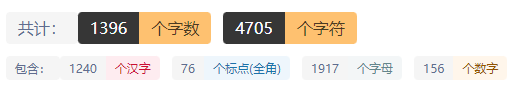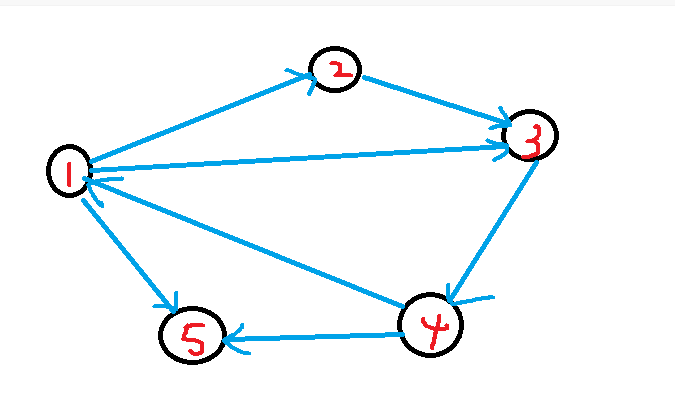# 建图基础¶

## 一、前言¶

### 1.1配套视频¶

https://www.bilibili.com/video/BV1RD4y1F7Fq

## 二、图的基本概念名词¶

• 在有向图中，箭头是具有方向的，从一个顶点指向另一个顶点，这样一来，每个顶点 被指向 的箭头个数，就是它的入度。从这个顶点指出去的箭头个数，就是它的出度

## 三、邻接矩阵（稠密图）¶

$$mp[i][j]$$表示地是$$i$$这个点指向j这个点的时候地边的权值

## 四、邻接表（稀疏图）¶

### 4.1使用容器vector¶

struct Edge{
int v,w;//v表示的是终点、w表示的是起点到重点的权值
};
vector<Edge>E[N];//这个N是根据你的顶点的大小来决定的


### 4.2使用原生数组¶

struct Edge{
int v,w;
struct Edge* next;
};
Edge E[N];


## 五、链式前向星¶

### 5.1前向星¶1 2

2 3

3 4

1 3

4 1

1 5

4 5

head len
head = 1 len = 3
head = 4 len = 1
head = 5 len = 1
head = 6 len = 2

### 5.2链式前向星¶

struct Edge{
int last;
int to;
int w;
};


void add(int u,int v,int w)
{
edge[cnt].w = w;//更改边权
edge[cnt].to = v;//更改下一个点的位置
}


edge.to = 2 edge.last = -1 head = 0;
edge.to = 3 edge.last= -1 head = 1
edge.to = 4 edge,last = -1 head = 2
edge.to = 3 edge.last = 0 head = 3
edge.to = 1 edge.last = -1 head = 4
edge.to = 5 edge.last= 3 head = 5
edge.to = 5 edge.last = 4 head = 6

for(int i=head[u];i!=-1;i=edge[i].last)